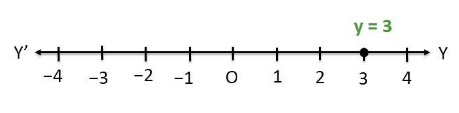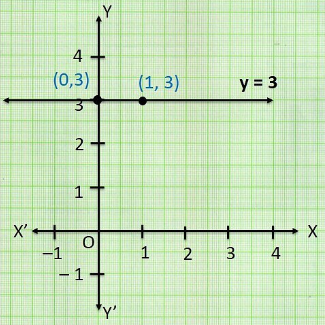Newbie

# Give the geometric representations of y = 3 as an equation (i) in one variable (ii) in two variables .Q.1

• 0

This is very important question of ncert class 9th of chapter linear equations in two variables  . How I solve the best solution of exercise 4.4 question number 1. Please help me to solve this in a easy and best way. Sir please help me to solve this question in a easiest method.

Share

1. Solution:

1. In one variable, y = 3(ii) In two variable, 0x+y = 3

When x = 0, y = 3

When x = 1, y = 3• 0